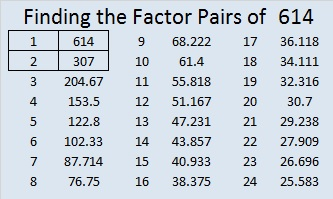614 and Level 6

I learned from OEIS.org that 614 is the smallest integer that can be expressed as the sum of 3 squares 9 different ways. I decided to see if I could find those 9 ways. Here they are:Print the puzzles or type the solution on this excel file: 12 Factors 2015-09-07

—————————————————————————————————

• 614 is a composite number.
• Prime factorization: 614 = 2 x 307
• The exponents in the prime factorization are 1 and 1. Adding one to each and multiplying we get (1 + 1)(1 + 1) = 2 x 2 = 4. Therefore 614 has exactly 4 factors.
• Factors of 614: 1, 2, 307, 614
• Factor pairs: 614 = 1 x 614 or 2 x 307
• 614 has no square factors that allow its square root to be simplified. √614 ≈ 24.77902—————————————————————————————————One thought on “614 and Level 6”

This site uses Akismet to reduce spam. Learn how your comment data is processed.